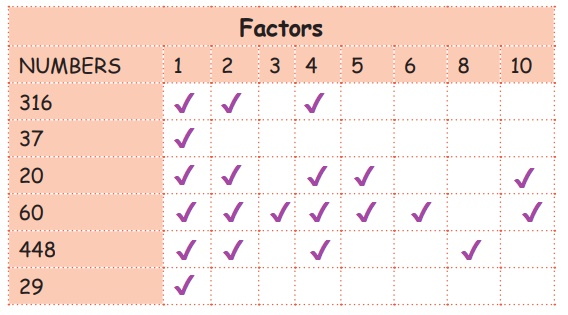Home | | Maths 5th Std | Composite and Prime Numbers

# Composite and Prime Numbers

A natural number having more than 2 factors is called a composite number. A natural number greater than 1, having only two factors namely one and the number itself is called a prime number.

Composite and Prime Numbers

Activity

Tick the factors of the following numbersComposite Number:

A natural number having more than 2 factors is called a composite number.

Examples

4, 6, 8, 9, 12, 26, 60, 448, 816,…

Prime number:

A natural number greater than 1, having only two factors namely one and the number itself is called a prime number.

Do you know? 2 is the only even prime number.

Examples

2, 3, 5, 7, 11, 13, 29, 37,….

COMMON FACTORS:

The factors that are common to two or more numbers is called common factors.

Example

The factors of 12, 18 are :

The factors of 12 - 1, 2, 3, 4, 6, 12

The factors of 18 - 1, 2, 3, 6, 9, 18The common factors of 12, 18 are 1, 2, 3 and 6.

ActivityTags : Numbers | Term 2 Chapter 2 | 5th Maths , 5th Maths : Term 2 Unit 2 : Numbers
Study Material, Lecturing Notes, Assignment, Reference, Wiki description explanation, brief detail
5th Maths : Term 2 Unit 2 : Numbers : Composite and Prime Numbers | Numbers | Term 2 Chapter 2 | 5th Maths